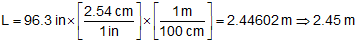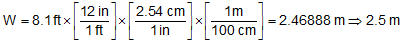## 5. Math operations

Measurements are often combined with other measurements to compute different quantities. It is sometimes a challenge to determine how many sig fig there should be in the computed quantity. To help, we have a few rules.

The result has the same level of accuracy as the least accurate number. The number of sig fig in the result is independent of the number of sig fig in any of the addends.

Example: What is the sum of 56.35 + 105 + 0.25 + 256.35

The raw sum is 471.95

The least accurate number is 105 which is good to the nearest 1

Therefore, the sum expressed to the nearest 1 is 472

Think of it this way: It's about 154 miles from where I'm sitting to Miller Field in Milwaukee. If I move my chair one foot further away, I'm still about 154 miles away from Miller Field. I wouldn't say Miller Field is about 154 miles and one foot away. I can't can state a result any more accurately than my least accurate number.

#### b. Multiplication & Division

The result has the same number of sig fig as the least sig fig in either of the numbers being multiplied or divided.

Example: What is the product of 46.53 x 23.1?

The raw product is 1074.843

46.53 has 4 sig fig, 23.1 has 3 sig fig

Therefore, the product expressed to 3 sig fig is 1070

Note the left 0 is significant, the right 0 is not

Example: convert 2.54 acres to square feet

There are 43,560 sq ft in 1 acre; this is an exact conversion factor

2.54 acres x (43,560 sq ft / 1 ac) = 110,642.4 sq ft (raw)

Since 2.54 has 3 sig fig, the area is 111,000 sq ft

#### c. Chain calculations

Chain calculations use combinations of the add/subtract and multiply/divide rules. Intermediate values are often rounded when performing long calculations, but doing so can have a cumulative effect on the final answer. To minimize this effect, intermediate values should be carried with extra digits and rounding done just once- at the end.

Example 1

What is the volume in cubic meters of the cube shown in Figure B-2?Figure B-2 Volume of a Cube

Volume of a cube is:Metric conversion factor (international foot definition, exact): 2.54 cm = 1 in

Method 1

Convert each dimension to meters, each expressed to correct number of sig fig.

Exact conversion factors are shown in brackets [].

L=96.3 in ←  3 sig figW=8.1 ft ← 2 sig figH=2.42 yd  3 sig figCompute the volumeSince width, W, only has 2 sig fig, the volume is 14 m3

Method 2

Same as Method 1 except carry an additional sig fig for intermediate calculations.

From the previous computations: L=2.446 m; W=2.47 m; H=2.213 m

Compute the volumeSince width, W, only has 2 sig fig, the volume is 13 m3

Carrying an additional sig fig for the intermediate calculations minimizes the cumulative rounding error. In this case, it affects the volume by one cubic meter (more than 7%).

Example 2

Convert 159°10'13" to decimal degrees with the correct number of sig fig.

This is an example of a single quantity (an angle) expressed in mixed units (deg, min, and sec). The initial inclination is to say there are 7 sig fig in the angle: 3 from the degrees plus 2 from the minutes plus 2 from the seconds. However that is incorrect.

• There are an infinite number of sig fig in the degrees portion - the angle contains exactly 159°. Any deviation from that is taken up in the minutes and seconds.
• There are an infinite number of sig fig in the minutes portion - the angle contains exactly 10'. Any deviation from that is taken up in the seconds.
• There are 2 sig fig in the seconds portion - it is the smallest unit and absorbs all the uncertainty in the degrees and minutes.

So how do we correctly convert mixed units to a single unit system?

Begin by converting all units to the smallest unit, the one subject to error: convert degrees and minutes to secondsThe conversion factors are exact so 572,400" and 600" are exact.

Add the angles part together in the smallest unit.Because the deg and min are exact, the least accurate number is 13", good to the nearest second. That makes the sum good to 6 sig fig based on the addition rule.

Convert the sum to the final unitsThe conversion factor is exact. The final result should have the same number of sig fig as the sum from the previous step.

The angle correctly converted is 159.170° to 6 sig fig.